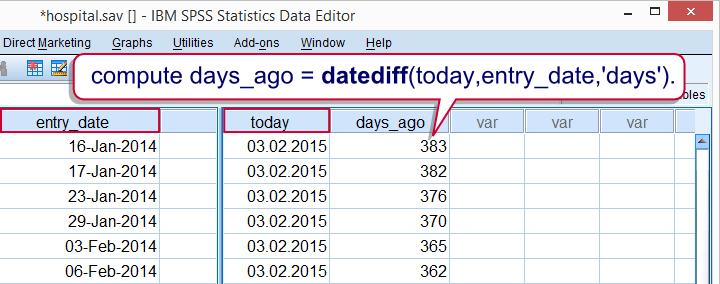SPSS TUTORIALS BASICS ANOVA REGRESSION FACTOR CORRELATION

# SPSS Date Variables Tutorials## SPSS Date Calculations

### SPSS Date Variables Basics

SPSS date variables are not hard to work with if you understand some basics. This tutorial walks you through them by showing some simple data examples.

### SPSS Date Calculations – A Quick Tutorial

SPSS date calculations are much easier than they may seem at first. The first key to success is understanding what SPSS date variables really are. Next, choose (and possibly combine) the right date functions. This tutorial will walk you through the main ones.

## SPSS Date Conversions

### Convert Numeric to Date Variable

For time calculations (such as the number of days between two dates) proper date variables are needed. In some cases, the digits of a numeric variable may represent year, month and day. This tutorial shows how to convert this format into an actual date variable.

### Extract a Year from a Date

Extracting the year (or any component) from a date is straightforward with the XDATE function.

## SPSS Date FAQ

### SPSS Computes Wrong Week Numbers?

SPSS computes wrong weeknumbers: some weeks consist of just 1 day. There seems to be no solution for computing correct ISO week numbers.

### SPSS – Convert String into Date Variable

For converting string variables to date variables, ALTER TYPE is the way to go. This tutorial demonstrates how to do this and points out a couple of caveats. For those who can't use ALTER TYPE, we'll propose some alternatives.

### Convert String Date to SPSS Date Variable

Converting a string variable into an SPSS date variable isn’t hard if you combine the right tools. Step-by-step tutorial with downloadable data file.

## SPSS Time Calculations

### SPSS Time Variables Basics

Working efficiently with SPSS time variables is not hard if you understand some basics. This tutorial walks you through just those.

### SPSS Time Variables Tutorial

Having a basic understanding of what SPSS time variables are, you may find calculations on them surprisingly easy. This tutorial will demonstrate SPSS' main time functions. However, we'll also show that we often don't even need them for getting things done.

## SPSS Datetime Calculations

### SPSS Datetime Variables Basics

Working with SPSS datetime variables is not hard at all if you understand some basics. This tutorial walks you through just those.

### SPSS Datetime Variables Tutorial

SPSS datetime variables: a must-read tutorial on working easily with them. Everything you want to get done demonstrated on real world data.

# Tell us what you think!

*Required field. Your comment will show up after approval from a moderator.

# THIS TUTORIAL HAS 3 COMMENTS:

•### By Amy on June 20th, 2018

14th October 1582 is the exact timepoint that SPSS uses to calculate it's date in seconds from. Just in case anyone wants to recode a date variable using syntax. You will need this date.

•### By Ruben Geert van den Berg on June 20th, 2018

Hi Amy!

If you'd like to RECODE a date variable, you could do so by recoding the underlying numbers which you'll see after running something like

FORMATS mydate(f16).

However, the more likely solution for this is using DATE.DMY combined with IF as in

IF(mydate > DATE.DMY(1,1,2018)) outputvariable = ....

For recoding date ranges, try IF with RANGE with DATE.DMY.

Hope that helps!

•### By Amy on July 3rd, 2018

Thanks Ruben! Helpful stuff - you really are a bit of an SPSS guru ;)